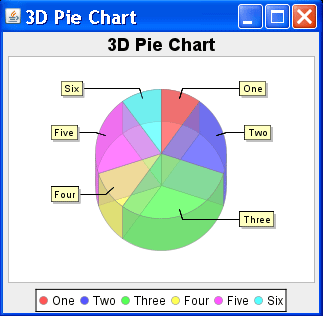Tutorials

# 3D Pie Chart

In this section we are providing you an example to create a 3D Pie Chart.

In this section we are providing you an example to create a 3D Pie Chart.

# 3D Pie Chart

In this section we are providing you an example to create a 3D Pie Chart.

Description of Program :

For creating a 3D Pie Chart we use PieDataset. In this example, for creating a PieDataset we are using DefaultPieDataset class. Then we add some data in this dataset that is shown in chart.

Description of Code :

For defining a dataset for a 3D Pie Chart we have to create an object of DefaultPieDataSet type :
DefaultPieDataset pieDataset = new DefaultPieDataset();

After creating the instance of dataset then we have to add the data in the data set by invoking the method setValue().
setValue(?one?, new Integer(10));

JFreeChart chart = ChartFactory.createPieChart3D("3D Pie Chart", pieDataset, true, true, true);
After added the data in dataset we create the 3D Pie Chart by invoking the createPieChart3D() method. This method is a static method of ChartFactory class and its returns the object of JFreeChart type. This method syntax is:
Public static JFreeChart createPieChart3D(java.lang.String title, PieDataset dataset, boolean legend, boolean tooltips, boolean urls)

PiePlot3D p=(PiePlot3D)chart.getPlot();
Above method is used to get the object of the plot for 3D Pie Chart. For this we have to invoke JfreeChart class method getPlot(). It returns the reference of Plot but we have to typecast it as a PiePlot3D.

p.setForegroundAlpha(0.5f);
Above method is used to set the alpha-transparency for the plot. It takes the float type argument.

ChartFrame frame1=new ChartFrame("3D Pie Chart",chart);
After this we create the object of ChartFrame. It used to display a chart.

Here is the code the of Program :

 ``` import java.awt.*; import org.jfree.chart.*; import org.jfree.chart.title.*; import org.jfree.data.general.DefaultPieDataset; import org.jfree.ui.*; import org.jfree.chart.plot.*; import org.jfree.util.*; public class Pie3D{ public static void main(String arg[]){   DefaultPieDataset pieDataset = new DefaultPieDataset();   pieDataset.setValue("One", new Integer(10));   pieDataset.setValue("Two", new Integer(20));   pieDataset.setValue("Three", new Integer(30));   pieDataset.setValue("Four", new Integer(10));   pieDataset.setValue("Five", new Integer(20));   pieDataset.setValue("Six", new Integer(10));   JFreeChart chart = ChartFactory.createPieChart3D   ("3D Pie Chart", pieDataset, true,true,true);   PiePlot3D p=(PiePlot3D)chart.getPlot();   p.setForegroundAlpha(0.5f);   ChartFrame frame1=new ChartFrame("3D Pie Chart",chart);   frame1.setVisible(true);   frame1.setSize(300,300);   } }```

Output of the Program :